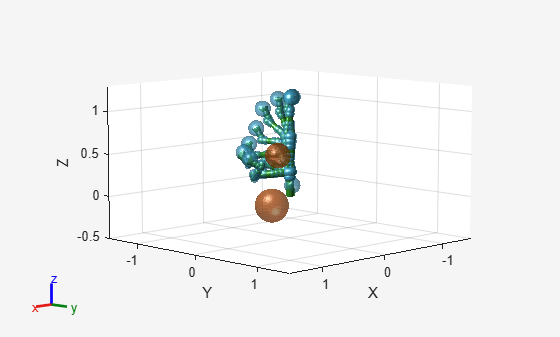# manipulatorCHOMP

Covariant Hamiltonian optimizer for rigid body tree motion planning

Since R2023a

## Description

The `manipulatorCHOMP` object optimizes rigid body tree motions using the Covariant Hamiltonian Optimization for Motion Planning (CHOMP) algorithm. CHOMP optimizes trajectories for smoothness and collision avoidance by minimizing a cost function comprised of a smoothness cost and collision cost.

To specify options for smoothness cost, collision cost, and the solver, use the `chompSmoothnessOptions`, `chompCollisionOptions`, and `chompSolverOptions` objects, respectively.

## Creation

### Syntax

``manipCHOMP = manipulatorCHOMP(robotRBT)``
``manipCHOMP = manipulatorCHOMP(robotRBT,Name=Value)``

### Description

example

````manipCHOMP = manipulatorCHOMP(robotRBT)` creates a CHOMP-based optimizer for a rigid body tree `robotRBT`. The `robotRBT` argument sets the `RigidBodyTree` property.```
````manipCHOMP = manipulatorCHOMP(robotRBT,Name=Value)` specifies properties using one or more name-value arguments.```

## Properties

expand all

Smoothness cost options, specified as a `chompSmoothnessOptions` object.

By default, this property contains a `chompSmoothnessOptions` object with default property values.

Collision cost options, specified as a `chompCollisionOptions` object.

By default, this property contains a `chompCollisionOptions` object with default property values.

Motion-planning solver options, specified as a `chompSolverOptions` object.

By default, this property contains a `chompSolverOptions` object with default property values.

Robot model used for motion planning, specified as a `rigidBodyTree` object at object construction.

You can access the spherical approximation in `RigidBodyTreeSpheres` as collision geometries on the tree by using the `RigidBodyTree` property.

Spheres of the bodies in the rigid body tree, stored as a table. The table contains two columns. The first column contains the name of a rigid body in `RigidBodyTree` and the second column contains a corresponding cell array. Each cell array contains a 4-by-N matrix as the only element in the cell array, where N is the number of spheres for the corresponding rigid body. Each column of the matrix of spheres contains the information for a sphere in the form [`r`; `x`; `y`; `z`], defined with respect to the frame of the rigid body. `r` is the radius of the sphere, and `x`, `y`, and `z` are the `x`-, `y`-, and `z`-coordinates of the center of the sphere, respectively.

Spherical obstacles in the environment, specified as a 4-by-N matrix. Each column represents the information about each sphere in the form [`r`; `x`; `y`; `z`], defined with respect to the base frame of the robot. `r` is the radius of the sphere, and `x`, `y`, and `z` are the `x`-, `y`-, and `z`-coordinates of the center of the sphere, respectively.

## Object Functions

 `optimize` Optimize trajectory using CHOMP `show` Visualize CHOMP trajectory of rigid body tree

## Examples

collapse all

Load a robot model into the workspace, and create a CHOMP solver.

```robot = loadrobot("kinovaGen3",DataFormat="row"); chomp = manipulatorCHOMP(robot);```

Create spheres to represent obstacles, and add them to the CHOMP solver.

```env = [0.20 0.2 -0.1 -0.1; % sphere, radius 0.20 at (0.2,-0.1,-0.1) 0.15 0.2 0.0 0.5]'; % sphere, radius 0.15 at (0.2,0.0,0.5) chomp.SphericalObstacles = env;```

To prioritize a collision-free trajectory, set the smoothness cost weight to a lower value than the collision cost weight. Then add the options to the CHOMP solver.

```chomp.SmoothnessOptions = chompSmoothnessOptions(SmoothnessCostWeight=1e-3); chomp.CollisionOptions = chompCollisionOptions(CollisionCostWeight=10); chomp.SolverOptions = chompSolverOptions(Verbosity="none",LearningRate=7.0);```

Initialize a trajectory, optimize it using the CHOMP solver, and show the waypoints in a figure.

```startconfig = homeConfiguration(robot); goalconfig = [0.5 1.75 -2.25 2.0 0.3 -1.65 -0.4]; timepoints = [0 5]; timestep = 0.1; trajtype = "minjerkpolytraj"; [wptsamples,tsamples] = optimize(chomp, ... [startconfig; goalconfig], ... timepoints, ... timestep, ... InitialTrajectoryFitType=trajtype); show(chomp,wptsamples,NumSamples=10); zlim([-0.5 1.3])```Ratliff, Nathan, Siddhartha Srinivasa, Matt Zucker, and Andrew Bagnell. “CHOMP: Gradient Optimization Techniques for Efficient Motion Planning.” In 2009 IEEE International Conference on Robotics and Automation, 489–94. Kobe, Japan: IEEE, 2009. https://doi.org/10.1109/ROBOT.2009.5152817.